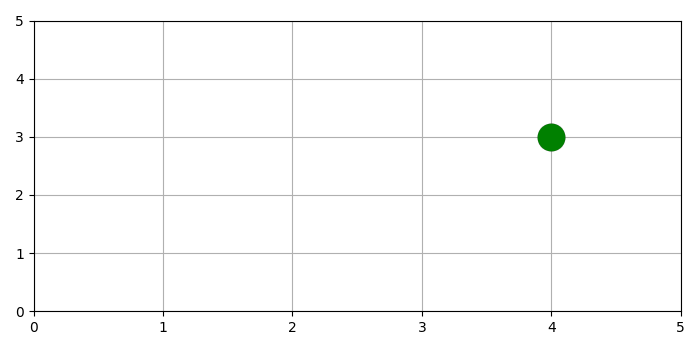# How to remove the outline of a circle marker when using pyplot.plot in Matplotlib?

To remove the outline of a circle marker, we can reduce the value of marker edge width.

• Initialize list for and y, with a single value.

• Limit and axis range for 0 to 5.

• Lay out a grid in current line style.

• Plot the given and using plot() method, with marker="o", markeredgecolor="red", markerfacecolor="green" and minimum markeredgewidth to remove the outline.

• To display the figure, use show() method.

## Example

from matplotlib import pyplot as plt
plt.rcParams["figure.figsize"] = [7.00, 3.50]
plt.rcParams["figure.autolayout"] = True
x = 
y = 
plt.xlim(0, 5)
plt.ylim(0, 5)
plt.grid()
plt.plot(x, y, marker="o", markersize=20, markeredgecolor="black", markerfacecolor="green", markeredgewidth=.1)
plt.show()

## OutputUpdated on: 06-May-2021

640 Views